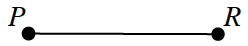### Home > INT2 > Chapter 7 > Lesson 7.1.5 > Problem7-65

7-65.Multiple Choice: Ziva is using a compass and a straightedge to construct the midpoint $M$ of line segment $\overline{PR}$. What is her first step?

1. Place the point of the compass on point $P$ and draw an arc halfway between points $P$ and $R$.

2. Use a straightedge to measure $PR$ and divide that measurement by $2$ to calculate $PM$.

3. Place the point of the compass on point $P$ and draw arcs above and below $PR$ using the same compass setting.

4. Fold the paper so that points $P$ and $R$ coincide.

C